# MCAT Physical : Orbitals and Hybridization

## Example Questions

### Example Question #1 : Orbitals And Hybridization

Electronegativity is an important concept in physical chemistry, and often used to help quantify the dipole moment of polar compounds. Polar compounds are different from those compounds that are purely nonpolar or purely ionic. An example can be seen by contrasting sodium chloride, NaCl, with an organic molecule, R-C-OH. The former is purely ionic, and the latter is polar covalent.

When comparing more than one polar covalent molecule, we use the dipole moment value to help us determine relative strength of polarity. Dipole moment, however, is dependent on the electronegativity of the atoms making up the bond. Electronegativity is a property inherent to the atom in question, whereas dipole moment is a property of the bond between them.

For example, oxygen has an electronegativity of 3.44, and hydrogen of 2.20. In other words, oxygen more strongly attracts electrons when in a bond with hydrogen. This leads to the O-H bond having a dipole moment.

When all the dipole moments of polar bonds in a molecule are summed, the molecular dipole moment results, as per the following equation.

Dipole moment = charge * separation distance

A single polar bond, such as the O-H bond described in the passage, is made up of two shared electrons. Before they join together in a bond, what subshell corresponds to the second quantum number (L) of the highest energy valence electrons of oxygen and hydrogen, respectively?

s and s

s and p

p and s

p and p

d and s

p and s

Explanation:

The highest energy electrons are those that are in the orbitals most removed from "s," in the order of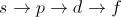.

As you add electron shells (n = 1 to n =2 to n = 3 and so on), you add one more of these orbitals. The result is that hydrogen only has an "s" orbital because it has n = 1, but oxygen has "s" and "p" because it has n = 2.

### Example Question #2 : Orbitals And Hybridization

Consider the following combustion reaction.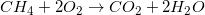What is the hybridization of carbon as a reactant? What is the hybridization of carbon as a product?

Reactant carbon configuration: sp3

Product carbon configuration: sp2

Reactant carbon configuration: sp3

Product carbon configuration: sp3

Reactant carbon configuration: sp

Product carbon configuration: sp3

Reactant carbon configuration: sp3

Product carbon configuration: sp

Reactant carbon configuration: sp3

Product carbon configuration: sp

Explanation:

In order to find the hybridization of an atom, simply count the number of sigma bonds and lone electron pairs around the atom. The s subshell is filled first, followed by the three p subshells.

Carbon as a reactant (methane) has four hydrogens around it, giving it four bonds. As a result, it displays sp3 hybridization.

As a product (carbon dioxide), carbon is attached to two oxygen atoms. These two sigma bonds give the carbon sp hybridization.

### Example Question #3 : Orbitals And Hybridization

Fundamental, or f orbitals, have shapes that are quite complex and difficult to draw. How many suborbitals are there in the f block?

Seven

Five

One

Two

Three

Seven

Explanation:

The f orbital is expressed in the lanthanides and the actinides. These groups contain fourteen elements in each row. Since each suborbital can contain two electrons, the correct answer is fourteen divided by two, or seven.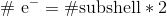The trend for suborbitals is determined by the l quantum number. The number of possible values for l determine the number of subshells.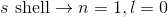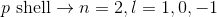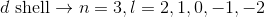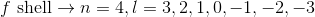### Example Question #62 : General Chemistry

How many electrons can fit in the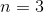electron shell?

Ten

Fourteen

Six

Eight

Eighteen

Eighteen

Explanation:

Anshell contains 3s, 3d, and 3p subshells. Any s shell can hold up to two electrons, any p shell can hold six electrons, and any d shell can hold ten electrons.

This question is best solved by using quantum numbers. We are given the principle quantum number. Using this, we can work down to determine how many electrons can fit in this shell. The rules for quantum numbers are given below.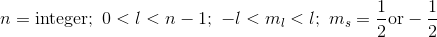If the principle quantum number is three, then the next quantum number can be zero, one, or two. These correspond to the s, p, and d subshells, respectively.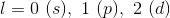After that, the next quantum number describes the orbitals within the subshells. Each orbital can carry two electrons, according to the final quantum number.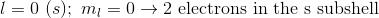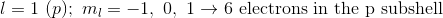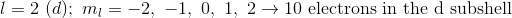In total, there are eighteen electrons allowed in all subshells of the third energy level.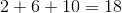### Example Question #4 : Orbitals And Hybridization

When writing the electron configuration for a 4p subshell, a student places two electrons in the first orbital before placing any in the other two. Which fundamental concept is the student forgetting?

Hund’s rule

Heisenberg’s uncertainty principle

Newton’s second law

Pauli exclusion principle

VESPR rules

Hund’s rule

Explanation:

Hund's rule states that every orbital in a subshell must be half-filled (occupied by one electron) before a second electron is added to any of them, thus, Hund's rule is the correct answer. The Pauli exclusion principle states that no two electrons may have the same four quantum numbers, and Heisenberg's uncertainty principle says that we cannot simultaneously know the position and momentum of a subatomic particle. Newton's laws and VSEPR rules have nothing to do with quantum mechanics.

### Example Question #5 : Orbitals And Hybridization

Which molecule has the shortest bond between two carbons?

Ethyne

Butane

Benzene

Ethene

Ethyne

Explanation:

Bond distance is related to the type of bond between the two atoms. The more bonds between two atoms, the shorter the distance. In other words, a triple bond is shorter than a double bond, and a double bond is shorter than a single bond. Ethyne is the only option that has a triple bond between the carbons, so it has the shortest bond distance.

### Example Question #6 : Orbitals And Hybridization

What is the electron configuration of chlorine?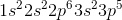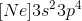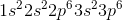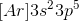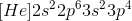Explanation:

Each period (row) of the periodic table corresponds to a principle quantum number, or electron energy shell. An electron is added for each element as one moves across the period. The first two electrons (groups 1 and 2) are placed in the s subshell. The next six electrons (groups 13 through 18) are placed in the p subshell.

Chlorine has an atomic number of 17, meaning that it will have a total of 17 electrons distributed through these shells and subshells. Start at the 1s subshell and work upward. Note that there is no 1p subshell.

Chlorine: 1s22s22p63s23p5

Another way of writing electron configuration for an element is to first write the noble gas that comes immediately before that element in brackets, and then write the rest of the notation. For example, the bracket notation for chlorine would be: [Ne]3s23p5